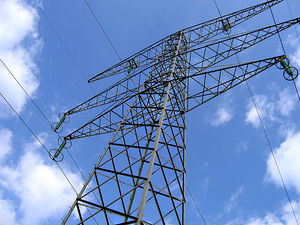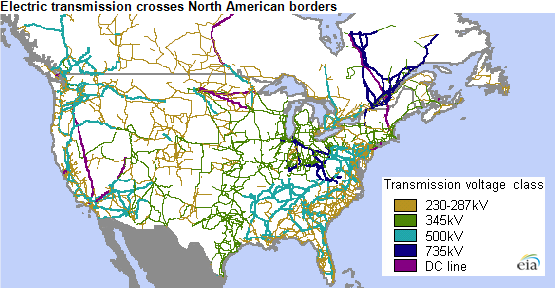# Electrical transmissionFigure 1. High voltage power lines are used for the transmission of electricity over long distances.

Electrical transmission is the process of delivering generated electricity - usually over long distances - to the distribution grid located in populated areas. An important part of this process includes transformers which are used to increase voltage levels to make long distance transmission feasible.

The electrical transmission system combined with power plants, distribution systems, and sub-stations to form what is known as the electrical grid. The grid meets society's electricity needs, and is what gets the electrical power from its generation to its end use. Since power plants are most often located outside of densely populated areas, the transmission system must be fairly large.

## Power Lines

Power lines or transmission lines, such as those in Figure 1, transport electricity from place to place. Usually, this electricity is alternating current so step-up transformers can increase the voltage. This increased voltage allows efficient transmission for 500 kilometers or less. There are 3 types of lines:

• Overhead lines are very high voltage, between 100 kV and 800 kV, and do the majority of long distance transmission. They must be high voltage in order to minimize power losses to resistance.
• Underground lines are used to transport power through populated areas, underwater, or pretty much anywhere that overhead lines can't be used. They are less common than overhead lines due to heat-related losses and higher cost.
• Subtransmission lines carry lower voltages (26 kV - 69 kV) to distribution stations, and can be overhead or underground.Figure 2. Transmission line map of USA and Canada.

### Reducing Power Line Losses

Power lines lose power to resistance, which is heat generated by moving electric current through a resistor. The loss of power () is given by the equation:

where

Above, it was mentioned that high voltage lines reduce this lost power. This fact can be explained by looking at the transmitted power, . As the voltage gets higher, the current must decrease in proportion because the power is remaining constant. For example, if the voltage is increased by a factor of 100, the current must decrease by a factor of 100 and the resulting power lost will be decreased by 1002 = 10000. However there is a limit, being that at extremely high voltages (2000 kV) the electricity begins to discharge resulting in high losses. In the transmission and distribution of electricity in the United States, the EIA estimates that about 6% of the electricity is lost.